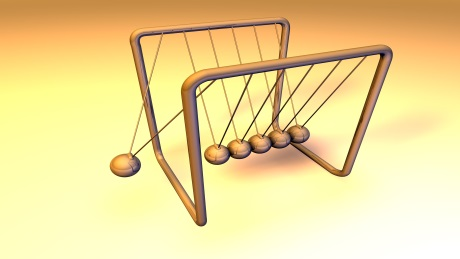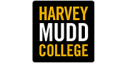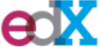FeedbackSave course to Your Buffet - Get notified, Track Progress, Plan Future Learning.
8 People Have this course in their Buffet

#### How Stuff Moves

Classified as: PHYS101 - Intro to Mechanics (5 Alt. Courses Available)
Course Description:

WHAT IS “HOW STUFF MOVES”? Mechanics is the study of how things move. It was the first quantitative science to achieve wide power to predict behavior, including things never before directly observed. Newton, Leibniz, and others invented calculus to describe motion and we will find both differential and integral calculus extremely useful throughout this course. This course parallels the second-semester mechanics course taught at Harvey Mudd College.  This course is an invitation to develop your problem-solving skills and to learn how to apply mathematics to all sorts of problems of the physical world. Learning the rules that govern how stuff moves in the world around us is exciting; using those rules to predict correctly something that you haven’t observed means that you really understand something. It‘s a great feeling. WHAT SHOULD I KNOW BEFORE WE START? You need not have taken physics before, but we assume that you have studied mathematics, including: algebra — including solving systems of 2 or 3 equations in as many unknown quantities, simplifying expressions, factoring, etc. trigonometry — the unit circle, definition of the standard trigonometric functions (sine, cosine, tangent, and their inverse functions), radian measure for angles, the law of cosines geometry — basic properties of circles, triangles, rectangles, parallel lines functions — if the x position of an object is a function of time t, how to graph x(t) given some functional form, and understanding that such an expression means that the x position has a unique value for all values of t [often in a finite range of times, to be sure] differential calculus — the meaning of the derivative of a function of a single variable and how to compute derivatives for common functions integral calculus — the meaning of the integral of a function, how to compute simple integrals using common strategies (substitution, including trigonometric substitution, parts, etc.) You may be taking a calculus course concurrently with this course; that should be a good strategy. We will introduce important calculus ideas and methods as the need arises and provide examples. There is a Mathematics Diagnostic Test that you can take to find out if your mathematics background is good to go.

• Instructor(s) Peter Saeta, Elizabeth Connolly
• University• Provider• Start Date 02/Feb/2015
• Duration Always Available
• Main Language English
Did you find any errors in this course listing ? Help us improve and we would be eternally grateful

#### Course Reviews

• No Comments Yet! Be the first one to comment.